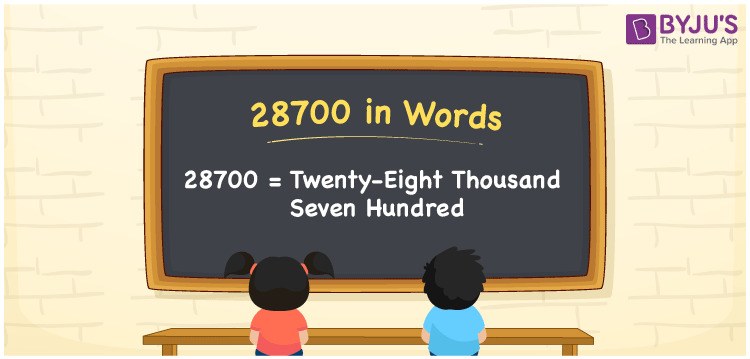# 28700 in Words

28700 in words is written as Twenty-eight thousand seven hundred. In both the International System of Numerals and the Indian System of Numerals, 28700 is written as Twenty-eight thousand seven hundred. The number 28700 is a Cardinal Number as it represents some quantity. For example, “that laptop costs 28700 rupees”.

 28700 in Words Twenty-eight thousand seven hundred Twenty-eight thousand seven hundred in Number 28700

## 28700 in English Words

28700 in English words is read as “Twenty-eight thousand seven hundred.”## How to Write 28700 in Words?

To write 28700 in words, we shall use the place value chart. In the place value chart, put 2 in the ten thousands, 8 in the thousands, 7 in the hundreds and put 0 in the rest. Let us make a place value chart to write the number 28700 in words.

 Ten Thousands Thousands Hundreds Tens Ones 2 8 7 0 0

Thus, we can write the expanded form as

2 × Ten Thousand + 8 × Thousand + 7 × Hundred + 0 × Ten + 0 × One

= 2 × 10000 + 8 × 1000 + 7 × 100 + 0 × 10 + 0 × 1

= 20000 + 8000 + 700 + 0 + 0

= 28700

= Twenty-eight thousand seven hundred.

28700 is a natural number, the successor of 28699 and the predecessor of 28701.

28700 in words – Twenty-eight thousand seven hundred

• Is 28700 an odd number? – No
• Is 28700 an even number? – Yes
• Is 28700 a perfect square number? – No
• Is 28700 a perfect cube number? – No
• Is 28700 a prime number? – No
• Is 28700 a composite number? – Yes

## Frequently Asked Questions on 28700 in Words

Q1

### How to write 28700 in words?

28700 in words is written as Twenty-eight thousand seven hundred.
Q2

### How to write 28700 in the International and Indian System of Numerals?

In both, the system of numerals, 28,700 in words, is written as Twenty-eight thousand seven hundred.
Q3

### How to write 28700 in a place value chart?

In the place value chart, write 2 in the ten thousands, 8 in the thousands, 7 in the hundreds and 0 in the tens and the ones, respectively.